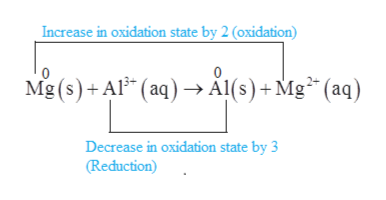# How many electrons are transferred in the following reaction? (The reaction is unbalanced.)Mg(s) + Al3+(aq)→Al(s) + Mg2+(aq)

Question
179 views
How many electrons are transferred in the following reaction? (The reaction is unbalanced.)
Mg(s) + Al3+(aq)→Al(s) + Mg2+(aq)
check_circle

Step 1

The given reaction is represented as follows:

Step 2

To calculate the number of electrons transferred in the reaction, the species undergoing oxidation and reduction should be identified first as follows:help_outlineImage TranscriptioncloseIncrease in oxidation state by 2 (oxidation) Mg (s)+Al (aq)> Al(s)+Mg* (aq) Decrease in oxidation state by 3 (Reduction) fullscreen
Step 3

According to the reaction, magnesium is getting oxidized by losing 2 electrons whereas aluminum is getting reduced by gaining 3 electrons. Thus, to balance the reaction the charges must be balanced.

Therefore, to bala...

### Want to see the full answer?

See Solution

#### Want to see this answer and more?

Solutions are written by subject experts who are available 24/7. Questions are typically answered within 1 hour.*

See Solution
*Response times may vary by subject and question.
Tagged in

### Chemistry